Thursday 04th June 2020
 CBSE Guess > Papers > Question Papers > Class XII > 2005 > Chemistry > Outside Delhi Set-I CHEMISTRY 2005 (Set-I Outside Delhi)
Q. 1. How many atoms can be assigned to its unit cell if an element forms (i) body centred cubic cell, and (ii) a face centred cubic cell? 1 Q. 2. What would be the value of Van't Hoff factor for a dilute solution of K 2 SO4 in water? 1 Q. 3. Express the relation between the half-life period of a reactant and its initial concentration for a reaction of nth order. 1 Q. 4. Mention a chemical property in which methanoic acid differs from acetic acid. 1 Q. 5. How is the basic strength of aromatic amines affected by the presence of an electron releasing group on the benzene ring? 1 Q. 6. State that de Broglie relationship. How do de Broglie waves of a moving particle differ from electromagnetic waves? 2 Or Show that the uncertainty principle is of little significance for an object of mass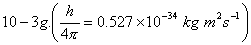Q. 7. Predict the products of electrolysis obtained at the electrodes in each case when the electrodes used are of platinum: 2 (i) An aqueous solution of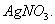(ii) An aqueous solution of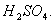Q. 8. State the basic reason of each of the following statements: 2 (i) InCI undergoes disproportionation reaction but TICI does not. (ii) AICI3 acts as a Lewis acid. Q. 9. Write chemical equations for the following reactions: 2 (i) Ca3 (PO4 )2 +SiO2 +C –> (ii) XeF6 +H2O –> Q. 10. Identify and mark the presence of centres of chirality, if any, in the following molecules. Mention the number of stereoisomers possible in each case. 2 (i) H3C - CH - CH 2 - CH - CH3                   |                      |                 OH                  OH (ii)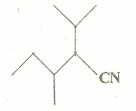Q. 11. Explain how an OH group attached to a carbon in the benzene ring activates benzene towards electrophilic substitution. 2 Q. 12. How are polymers classified on the basis of forces operating between their molecules? To which, of these classes does nylon-66 belong? 2 Q. 13. (a) Use the LCAO method for the formation of molecular orbitals in case of homonuclear diatomic hydrogen molecule. (b) Which of the following has higher bond dissociation energy and why? (i)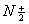(ii)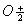3 Or What kinds of molecular forces exist between the species in the following pairs of particles and why? (i) He and N2 (ii) Cl2 and NO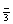(iii) NH3 and CO Q. 14. Aluminium crystallises in a face centred cubic close-packed structure its atomic radius is 125 x 10-12 m. (a) What is the length of the edge of the unit cell? (b) How many such unit cells are there in a 1.00 m piece of aluminium? 3 Q. 15. State Henry's law for solubility of a gas in a liquid Explain the significance of Henry's law constant ( At the same temperature, hydrogen Is more soluble in water than helium. Which of them will have a higher value of KH and why? 3 Q. 16. The activation energy of a reaction is 75.2 kJ mor -1 in the absence of a catalyst and 50.14 kJ mol-1 with a catalyst. How many times will the rate of reaction grow in the presence of the catalyst if the reaction proceeds at 250C? (R=8.3I4 JK-1 mol-1) 3 Q. 17. How do size of particles of adsorbent, pressure of gas and prevailing temperature influence the extent of adsorption of a gas on a solid? 3 Q. I8. (a) Write the structural formula of hex-2-en-4 ynoic acid. (b) To illustrate the following reactions write one chemical equation for each: (i) Cross aldol condensation (ii) Hofmann bromamide reaction 3 Q. 19. Write the chemical reaction equation stating the reaction conditions required for each of the following conversions: 3 (i) Methyl bromide to ethylamine (ii) Aniline to phenol (iii) p-toluidine to 2-bromo-4-methylanilline Q. 20. (a) Write the corresponding chemical reaction equation to show that (i) PbO2 can act as an oxidising agent. (ii) All the bonds in a molecule of PCI5 are not equivalent. (b) Write the structural formula for either XeF2 or IF3. 3 Q. 21. Draw a sketch to show the splitting of d-orbitals in an octahedral crystal field. State clearly how the actual configuration in split d-orbitals in an octahedral crystal field is decided by the magnitudes of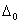and P values. 3 Q. 22. The E° values at 298 K corresponding to the following two reduction electrode processes are: (i) Cu+ /Cu=+0.52 V (ii) Cu2 +/Cu+=+0.16 V Formulate the galvanic cell for their combination. What will be the cell poltenti-al? Calculate the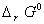for the cell reaction. (F=96500 C mol-1) 3 Q. 23. The radioactive isotope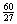Co, can be made by an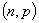or an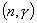nuclear reaction. State the appropriate target nucleus for each reaction. If the half-life ofCo is 7 years, how long will it take for complete annihilation and why? 3 Q. 24. Describe the following with an example each: 3 (i) Antimicrobials (ii) Acid dyes (iii) Antioxidants Q. 25. (a) The standard Gibbs energy change values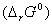at 1773 K are given for the following reactions: 4 Fe+3 O2 –> 2 Fe2 O3 ;G0 =-1487 kj mol-1 4 Al+3 O2 –> 2 Al2 O3 ;G0 =-22500 kj mol-1 2 CO+O2 –> 2 CO2 '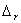G0 =-515 kj mol-1 Find out the possibility of reducing Fe2O3 and Al2O3 with CO at this temperature. (b) Comment on the following statements giving reasons: (i) An exothermic reaction is sometimes not spontaneous. (ii) Reactions withG0 values less than zero always have equilibrium constants greater than 1. 5 Or (a) The half-reactions are: (i) Fe3+ + e-- –> Fe2+ , E0 = 0.76 V (ii) Ag+ + e-- –> Ag, E0 = 0.80 V Calculate Kc for the following reaction at 25° C: Ag+ + Fe2+ –> Fe3+ +Ag (F=96500 C mol-1) (b) Define the following terms: (i) Isothermal and Adiabatic processes (ii) State variables / State functions Q. 26. (a) Given below are the electrode potential values, E° for some of the first row of transition elements: Element –>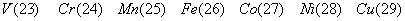E0 M2+ /M (v)=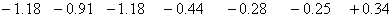Explain the irregularities in these values on the basis of electronic structures of atoms. (b) Complete the following reaction equations: 5 •  Cr2O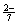+Sn2+ + H+ –> •  MnO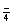+ Fe2+ + H+ –> Or (a) How would you account for the following: (i) Cobalt (II) is stable in aqueous solution. but in the presence of complexing reagents it is easily oxidised. (ii) The transition elements exhibit high enthalpy of atomization. (iii) Of the d4 species, Cr2+ is strongly reducing while Mn ( Ill ) is strongly oxidising. (b) Name the chief ore of copper and write the reactions Involved in its extraction from that ore. Q. 27. (a) Write the chemical reactions of glucose with (i) NH2 OH and (ii) (CH3 CO)2 o.Also draw simple Fischer projections of D-glucose and L-glucose. (b) Name the food sources and the deficiency diseases caused due to lack of any two of vitamins A, C, E and K.5 Or (a) State the composition and functional differences between DNA and RNA. Describe the mechanism of replication of DNA. (b) Define 'mutation'. 3, 2 English 2005 Question Papers Class XII Delhi Outside Delhi Set I Set I Set II Set II Set III Set III CBSE 2005 Question Papers Class XII